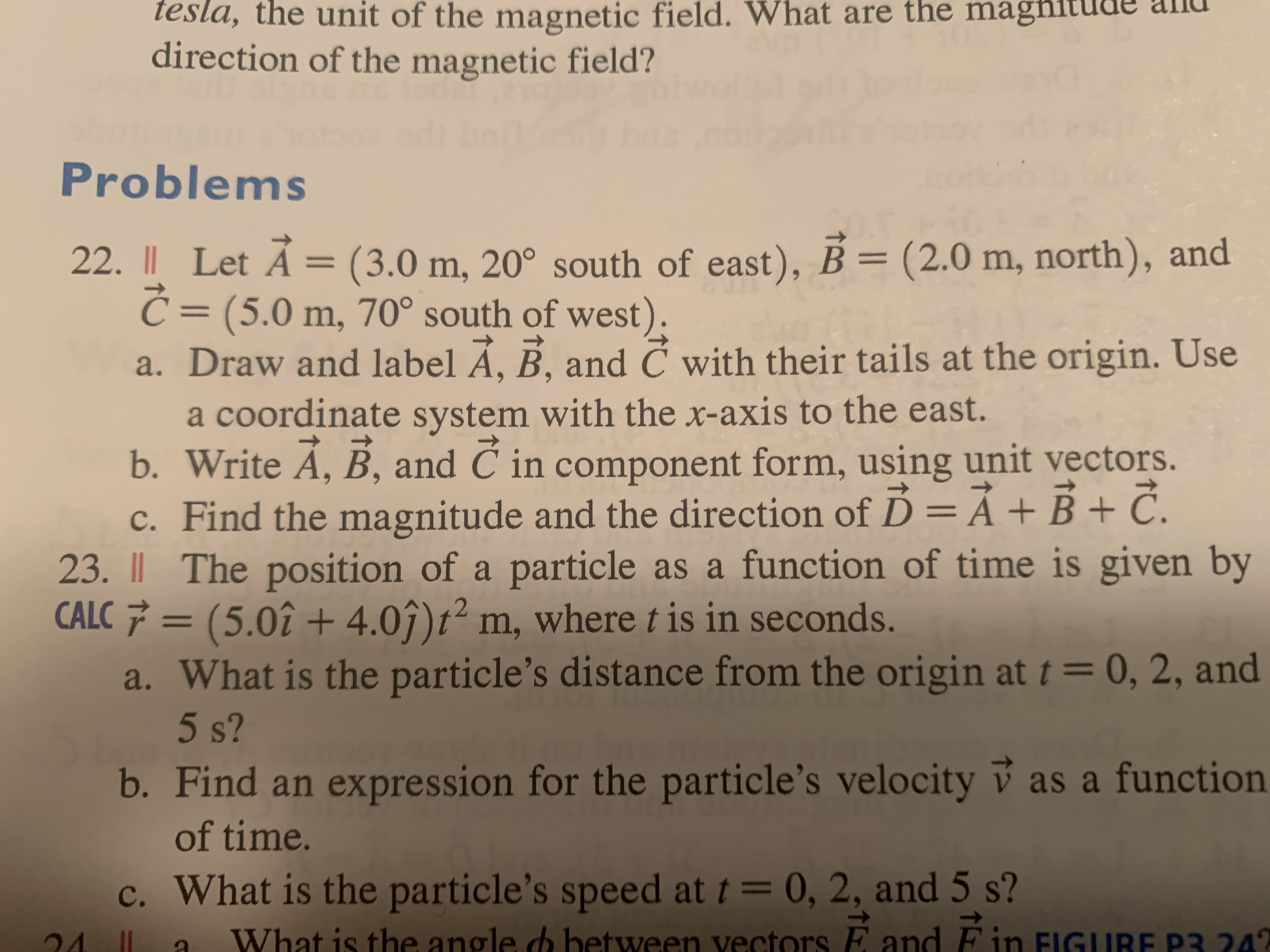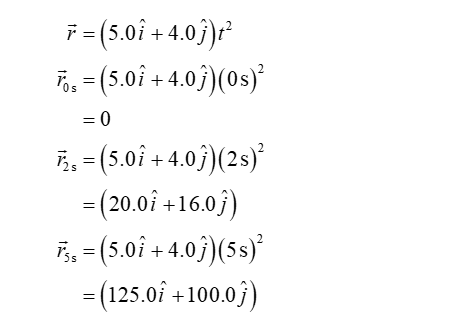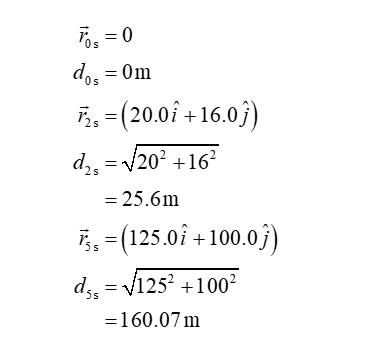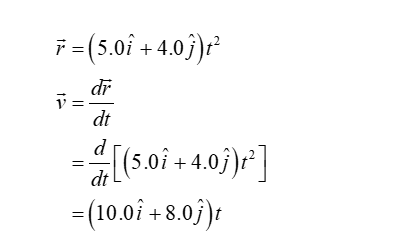# tesla, the unit of the magnetic field. What are the magħitude anddirection of the magnetic field?Problems22. || Let Á = (3.0 m, 20° south of east), B = (2.0 m, north), andĆ = (5.0 m, 70° south of west).a. Draw and label Á, B, and Č with their tails at the origin. Usea coordinate system with the x-axis to the east.b. Write A, B, and C in component form, using unit vectors.%3D%3D6.c. Find the magnitude and the direction of Ď =Ả+B + Č.23. I| The position of a particle as a function of time is given byCALC 7 = (5.0î + 4.0î)t² m, where t is in seconds.a. What is the particle's distance from the origin at t = 0, 2, and5 s?b. Find an expression for the particle's velocity v as a function%3Dof time.c. What is the particle's speed at t= 0, 2, and 5 s?FinWhat is the angle d between vectors EE and242

Question
1 views

The question is on number 23help_outlineImage Transcriptionclosetesla, the unit of the magnetic field. What are the magħitude and direction of the magnetic field? Problems 22. || Let Á = (3.0 m, 20° south of east), B = (2.0 m, north), and Ć = (5.0 m, 70° south of west). a. Draw and label Á, B, and Č with their tails at the origin. Use a coordinate system with the x-axis to the east. b. Write A, B, and C in component form, using unit vectors. %3D %3D 6. c. Find the magnitude and the direction of Ď =Ả+B + Č. 23. I| The position of a particle as a function of time is given by CALC 7 = (5.0î + 4.0î)t² m, where t is in seconds. a. What is the particle's distance from the origin at t = 0, 2, and 5 s? b. Find an expression for the particle's velocity v as a function %3D of time. c. What is the particle's speed at t= 0, 2, and 5 s? Fin What is the angle d between vectors E E and 242 fullscreen
check_circle

Step 1

a.

The position of particle at t = 0,2, and 5 s can be calculated asStep 2

Then the distance from the origin at t = 0,2, and 5 s can be calculated as follows,b.

The expression of the velocity for the given case is...

### Want to see the full answer?

See Solution

#### Want to see this answer and more?

Solutions are written by subject experts who are available 24/7. Questions are typically answered within 1 hour.*

See Solution
*Response times may vary by subject and question.
Tagged in

### Physics